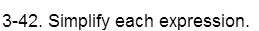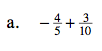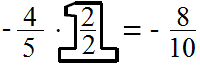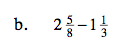### Home > MC2 > Chapter 3 > Lesson 3.1.4 > Problem3-42

3-42.Refer to 3-22 for help.

Find a common denominator.$-\frac{8}{10} + \frac{3}{10} = - \frac{5}{10} = -\frac{ 1}{2}$You can either separate the whole numbers from the fractions and find the differences separately, or convert both into fractions greater than one first. We will show you how to do the latter below.

Multiply the whole number by the denominator, then add the amount of the numerator to find a new numerator.

Find a common denominator and multiply each fraction by Giant Ones.

Simplify by subtracting the numerators.

Convert back to a mixed number.

$\frac{16 + 5}{8} - \frac{3+1}{3} = \frac{21}{8} - \frac{4}{3}$

$\frac{21}{8} \times \frac{3}{3} - \frac{4}{3} \times \frac{8}{8} = \frac{63}{24} - \frac{32}{24}$

$\frac{31}{24}$

$1 \frac{7}{24}$